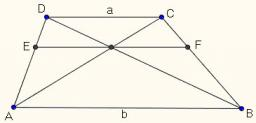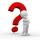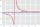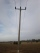# See harmonics

It is true that the size of the central segment of any trapezoid is the harmonic mean size of its bases? Prove it. Central segment crosses the intersection of the diagonals and is parallel to the bases.

ResultDid you find an error or inaccuracy? Feel free to write us. Thank you!Tips to related online calculators
Looking for calculator of harmonic mean?
Looking for a statistical calculator?

#### You need to know the following knowledge to solve this word math problem:

We encourage you to watch this tutorial video on this math problem:

## Related math problems and questions:

• Diagonal in rectangleIn that rectangle ABCD is the center of BC point E and point F is center of CD. Prove that the lines AE and AF divide diagonal BD into three equal parts.
• Trapezium diagonalsIt is given trapezium ABCD with bases | AB | = 12 cm, |CD| = 8 cm. Point S is the intersection of the diagonals for which |AS| is 6 cm long. Calculate the length of the full diagonal AC.
• Isosceles trapezoidIn an isosceles trapezoid KLMN intersection of the diagonals is marked by the letter S. Calculate the area of trapezoid if /KS/: /SM/ = 2:1 and a triangle KSN is 14 cm2.
• Diagonals at right angleIn the trapezoid ABCD, this is given: AB=12cm CD=4cm And diagonals crossed under a right angle. What is the area of this trapezoid ABCD?
• ReciprocalIt is true (prove it) that if a> b> 0: ?
• Diagonal intersectisosceles trapezoid ABCD with length bases | AB | = 6 cm, CD | = 4 cm is divided into 4 triangles by the diagonals intersecting at point S. How much of the area of the trapezoid are ABS and CDS triangles?
• Rectangular trapezoidThe ABCD rectangular trapezoid with the AB and CD bases is divided by the diagonal AC into two equilateral rectangular triangles. The length of the diagonal AC is 62cm. Calculate trapezium area in cm square and calculate how many differs perimeters of the
• Area of iso-trapFind the area of an isosceles trapezoid if the lengths of its bases are 16 cm and 30 cm, and the diagonals are perpendicular to each other.
• MO Z9–I–2 - 2017In the VODY trapezoid, VO is a longer base and the diagonal intersection K divides the VD line in a 3:2 ratio. The area of the KOV triangle is 13.5 cm2. Find the area of the entire trapezoid.
• MO - trianglesOn the AB and AC sides of the triangle ABC lies successive points E and F, on segment EF lie point D. The EF and BC lines are parallel and is true this ratio FD:DE = AE:EB = 2:1. The area of ABC triangle is 27 hectares and line segments EF, AD, and DB seg
• Trapezoid - intersection of diagonalsIn the ABCD trapezoid is AB = 8 cm long, trapezium height 6 cm, and distance of diagonals intersection from AB is 4 cm. Calculate the trapezoid area.
• Proof PTCan you easily prove Pythagoras theorem using Euclidean theorems? If so, do it.
• Two cablesOn a flat plain, 2 columns are erected vertically upwards. One is 7 m high and the other 4 m. Cables are stretched between the top of one column and the foot of the other column. At what height will the cables cross? Assume that the cables do not sag.
• Trapezoid IVIn a trapezoid ABCD (AB||CD) is |AB| = 15cm |CD| = 7 cm, |AC| = 12 cm, AC is perpendicular to BC. What area has a trapezoid ABCD?
• Trapezoid MO-5-Z8ABCD is a trapezoid that lime segment CE is divided into a triangle and parallelogram, as shown. Point F is the midpoint of CE, DF line passes through the center of the segment BE, and the area of the triangle CDE is 3 cm2. Determine the area of the trape
• Divide an isosceles triangleHow to divide an isosceles triangle into two parts with equal contents perpendicular to the axis of symmetry (into a trapezoid and a triangle)?
• ProveProve that k1 and k2 are the equations of two circles. Find the equation of the line that passes through the centers of these circles. k1: x2+y2+2x+4y+1=0 k2: x2+y2-8x+6y+9=0